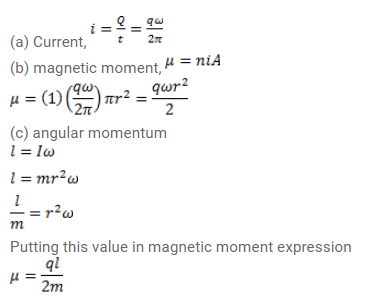# Consider a nonconducting ring of radius

Question:

Consider a nonconducting ring of radius $\mathrm{r}$ and mass $\mathrm{m}$ which has a total charge q distributed uniformly on it. The ring is rotated about its axis with an angular speed $\omega$. (a) Find the equivalent electric current in the ring, (b) find the magnetic moment $\mu$ of the ring. (c) Show that $\mu=\frac{q}{2 m} l$ where $\mid$ is the angular momentum of the ring about its axis of rotation.

Solution: